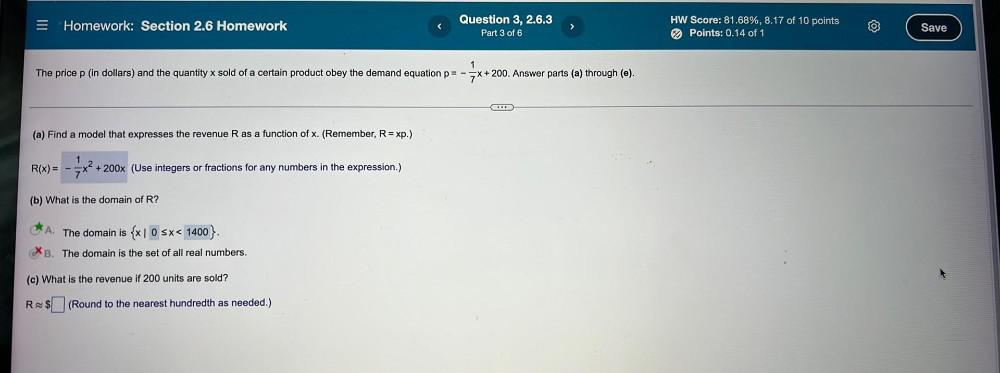Question:

# The price p (in dollars) and the quantity x sold of a certain product obey the demand equation p= -1/7x+200. Answer parts (a) through (e). (a) Find a model that expresses the revenue R as a function oThe price p (in dollars) and the quantity x sold of a certain product obey the demand equation p= -1/7x+200. Answer parts (a) through (e). (a) Find a model that expresses the revenue R as a function of x. (Remember, R = xp.) R(x) = -1/7x²+ 200x (Use integers or fractions for any numbers in the expression.) (b) What is the domain of R? A. The domain is {x l 0 ≤ x< 1400}. B. The domain is the set of all real numbers. (c) What is the revenue if 200 units are sold? R≈ \$□(Round to the nearest hundredth as needed.)